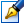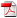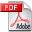##Lie algebras and invariant integrals for polynomial differential systems

 Author: Oxana Diaconescu Degree: doctor of physics and mathematics Speciality: 01.01.02 - Differential equations Year: 2008 Scientific adviser: Mihail Popadoctor habilitat, professor, Institute of Mathematics and Computer Science Institution: Institute of Mathematics and Computer Science Scientific council: DH 30-01.01.02-27.03.08 Moldova State University

### Status

The thesis was presented on the 25 July, 2008
Approved by NCAA on the 18 September, 2008

### Abstract0.47 Mb / in romanian

### Thesis

CZU 517.9252.25 Mb / in russian
127 pages

### Keywords

Lie algebra of operators; invariants, mixed comitants, contravariants and covariants of the n-dimensional differential system; invariant GL(n,R) -integral; functional base of center-affine invariants and comitants; integrating factor of differential system, differential system of Darboux type, first and particular integrals

### Summary

In the present thesis we consider a system of differential equations , (1) where are the coefficient tensors, symmetrical in lower indices, where the complete convolution holds, and is a finite set of real nonnegative numbers. In cases when , system (1) has absolute terms. As system (1) is well-defined by the number n and the set , later we will denote it as .

The work is devoted to application of Lie algebras of operators and of the theory of algebraic invariants to differential systems . An infinite-dimensional Lie algebra of operators, admitted by system , is considered. It is showed that this algebra has, as a subalgebra, a finite-dimensional Lie algebra, corresponding to the representation of center-affine group GL(n,R) in space of phase variables and coefficients of indicated system. Lie theorem on integrating factor is generalized for n-dimensional polynomial differential systems. There are constructed recurrent formulas of Lie operators, corresponding to one-parametrical elementary subgroups of group GL(n,R) in space of phase variables and coefficient of system . The functional basis of mixed comitants is established for system with respect to group GL(4,R). The absence of some dimensions of GL(n,R) -orbit is investigated for systems with n=4,5, and Lie algebra of operators, admitted by some canonical forms of indicated systems, is also constructed. An n-dimensional Lie algebra of operators is constructed and corresponds to the representation of group GL(n,R) in space of phase variables and coefficients of system with n=4,5, some its characteristics are investigated for n=4. With the aid of Lie algebras of operators, admitted by Darboux type's systems with m= , invariant GL(2,R) -integrating factors and invariant particular GL(2,R) -integrals were constructed. The invariant particular GL(2,R) -integrals in some certain cases are the limit cycles for indicated systems. It is showed that the class of two-dimensional polynomial differential systems (m>2), having the equality K2=0 as a center-affine quadratic integral, is wider then the class of Darboux type's systems, possessing this property. Moreover, obtained center-affine invariant conditions, ensuring this property, are necessary and sufficient conditions.

Some invariant GL(n,R) -integrals for affine systems with n=4,5 are considered. For Darboux type's system (n>2) there are constructed recurrent formulas for some invariant GL(n,R) -integrals. System is considered and its invariant GL(3,R) -integrals are found. For the same system, there are constructed examples with particular integrals, having the form of ellipsoid or sphere. Systems with n=4,5, with n=3,4 and are investigated in order to construct their invariant particular integrals.